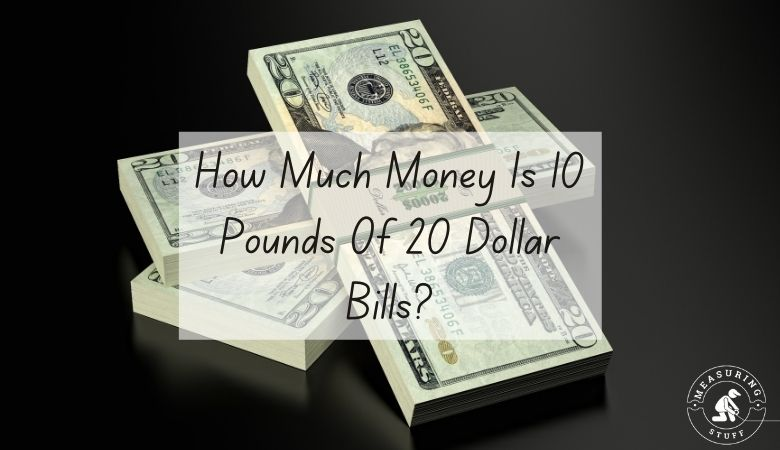# How Much Money Is 10 Pounds Of 20 Dollar Bills?

If you are trying to figure out how much money you would have with 10 pounds of 20 dollar bills, you are in the right place.

Most people are not lucky enough to have 10 pounds of 20 dollar bills, and you might think that you need to count each bill one by one to figure out the amount of money that you have.

Well, there is another way.

If you are able to weigh your bills accurately on a scale and determine that you have 10 pounds, you can use the formula below to easily come up with the answer. Remember to subtract the weight of the container or bag that the bills may be in.

So, how much money do you have with 10 pounds of 20 dollar bills?

The answer is you will have \$90,900. A single 20 dollar bill from the USA weighs 1 gram each which is 0.0022 pounds. Therefore 454 grams weigh 1 pound.

0.0022 x 454 = 1 pound.

To determine how much money is 10 pounds of 20 dollar bills, you just need to use a simple formula.

# of pounds in bills/weight per bill = # of bills.

In the example above, 10 pounds / 0.0022 = 4545.

Once you know how many 20 dollar bills you have, multiply that number by the value of the bill to tell you how much money you have.

4545 bills @ \$20 each = \$90,900.

4545 x 20 = 90,900.

Now you know that if you have a 10-pound stack of 20 dollar bills, you have exactly \$90,900.